# Vapor-liquid equilibrium

This is an attempt at describing how models can be written for solutions in vapor-liquid equilibrium. The answer is written in stages, to show how a model might reasonably be developed using ASCEND.

The first stage being all variables defined as a "factor". Variables are later defined as "pressure" or "temperature" and so on. While ASCEND allows the modeler to write without much/any structure, as the models become more complex and as the need for their distribution becomes greater, paying attention to the art of model writing becomes important.Yet, when a new user starts with the ASCEND documentation, it can be difficult to read through the elegant models. Thus, this exercise. Note that the discussion in the thin-walled tank tutorial details the type of thinking we use here in evolving a model.

The example ends with how P-x-y or T-x-y plots can be generated python and the scripts written by John Pye. The page was written by Krishnan Chittur.

## Question

This is Example 10.1 from the book Chemical Engineering Thermodynamics by Van Ness and Smith, 7th Edition, page 352. ISBN 0073104450 (read online with Amazon).

Binary system acetonitrile(1)/nitromethane(2) conforms closely to Raolt's law. Vapour pressures for the pure species are given by the following Antoine equations: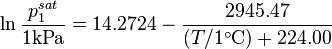$\ln \frac{p_1^{sat}}{1 \mathrm{kPa}} = 14.2724 - \frac{2945.47}{(T/{1^\circ \mathrm C)} + 224.00}$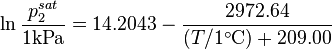$\ln \frac{p_2^{sat}}{1 \mathrm{kPa}} = 14.2043 - \frac{2972.64}{(T/{1^\circ \mathrm C)} + 209.00}$

Problem:

(a) Prepare a graph showing p vs x1 and p vs y1 for a temperature of 75 °C.

(b) Prepare a graph showing T vs x1 and T vs y1 for a pressure of 70 kPa.

A brief explanation of the variables. T is the system temperature, P is the total system pressure, x1,x2 are the mole fractions of the two components in the liquid phase and y1,y2 are the mole fractions of the two components in the vapor phase and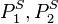$P_1^S, P_2^S$ are the vapor pressures of components 1 and 2 (which we assume we can predict using Antoine's equation and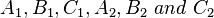$A_1, B_1, C_1, A_2, B_2~and~C_2$ are the Antoine's coefficients).

The relevant equations are (for a binary system)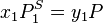$x_1 P_1^S = y_1 P$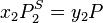$x_2 P_2^S = y_2 P$
x1 + x2 = 1.0
y1 + y2 = 1.0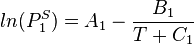$ln(P_1^S) = A_1 - \frac{B_1}{T + C_1}$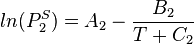$ln(P_2^S) = A_2 - \frac{B_2}{T + C_2}$

Name of Calculation What is Known What we want to calculate
Bubble Point Pressure T,x1 P,y1
Dew Point Pressure T,y1 P,x1
Bubble Point Temperature P,x1 T,y1
Dew Point Temperature P,y1 T,x1

### No Units, All variables declared as Factors

REQUIRE "atoms.a4l";

MODEL example101;

P1S, P2S, P, T, x1, x2, y1, y2 IS_A factor;

ln(P1S) = 14.2724 - 2945.47/(T + 224.0);

ln(P2S) = 14.2043 - 2972.64/(T + 209.0);

x1 + x2 = 1.0;
y1 + y2 = 1.0;

x1*P1S = y1*P;
x2*P2S = y2*P;

METHODS
METHOD parta;
FIX T; FIX x1;

x1 := 0.6;
T := 75.0;
END parta;

METHOD partb;
FIX P; FIX x1;

x1 := 0.1;
P := 70.0;
T := 300.0;

END partb;
METHOD doparta;
RUN ClearAll;

RUN parta;
END doparta;
METHOD dopartb;

RUN ClearAll;
RUN partb;
END dopartb;

END example101;


### Using an exponential function, instead of natural log (here's why)

REQUIRE "atoms.a4l";
MODEL example101;

P1S, P2S, P, T, x1, x2, y1, y2 IS_A factor;

P1S = exp(14.2724 - 2945.47/(T + 224.0));

P2S = exp(14.2043 - 2972.64/(T + 209.0));

x1 + x2 = 1.0;
y1 + y2 = 1.0;

x1*P1S = y1*P;
x2*P2S = y2*P;

METHODS
METHOD parta;
FIX T; FIX x1;

x1 := 0.6;
T := 75.0;
END parta;

METHOD partb;
FIX P; FIX x1;

x1 := 0.1;
P := 70.0;
T := 300.0;

END partb;
METHOD doparta;
RUN ClearAll;

RUN parta;
END doparta;
METHOD dopartb;

RUN ClearAll;
RUN partb;
END dopartb;

END example101;


### Constants in a METHOD called values

REQUIRE "atoms.a4l";
MODEL example101;

A1, B1, C1, A2, B2, C2 IS_A factor;

P1S, P2S, P, T, x1, x2, y1, y2 IS_A factor;

ln(P1S) = A1 - B1/(T + C1);

ln(P2S) = A2 - B2/(T + C2);

x1 + x2 = 1.0;
y1 + y2 = 1.0;

x1*P1S = y1*P;
x2*P2S = y2*P;

METHODS
METHOD values;
A1 := 14.2724; B1 := 2945.47; C1 := 224.0;

A2 := 14.2043; B2 := 2972.64; C2 := 209.0;

FIX A1; FIX B1; FIX C1; FIX A2; FIX B2; FIX C2;

END values;
METHOD parta;
FIX T; FIX x1;

x1 := 0.6;
T := 75.0;
END parta;

METHOD partb;
FIX P; FIX x1;

x1 := 0.1;
P := 70.0;
T := 300.0;

END partb;
METHOD doparta;
RUN ClearAll;

RUN values;
RUN parta;
END doparta;

METHOD dopartb;
RUN ClearAll;
RUN values;

RUN partb;
END dopartb;

END example101;


### Defining Units like Pressure and Temperature

REQUIRE "atoms.a4l";

MODEL example101;

A1, B1, C1, A2, B2, C2 IS_A factor;

P1S, P2S, P IS_A pressure;
T IS_A temperature;

x1, x2, y1, y2 IS_A fraction;

P1S/100000.0{Pa} = exp(A1 - B1/(T/1.0{K} - 273.15 + C1));

P2S/100000.0{Pa} = exp(A2 - B2/(T/1.0{K} - 273.15 + C2));

x1 + x2 = 1.0;
y1 + y2 = 1.0;

x1*P1S = y1*P;
x2*P2S = y2*P;

METHODS
METHOD default_self;
END default_self;
METHOD values;

A1 := 14.2724; B1 := 2945.47; C1 := 224.0;

A2 := 14.2043; B2 := 2972.64; C2 := 209.0;

FIX A1; FIX B1; FIX C1; FIX A2; FIX B2; FIX C2;

END values;

METHOD parta;
FIX T; FIX x1;

x1 := 0.6;
T := 348.15 {K};
END parta;

METHOD partb;
FIX P; FIX x1;

x1 := 0.1;
P := 70.0 {kPa};
END partb;

METHOD doparta;
RUN ClearAll;
RUN values;

RUN parta;
END doparta;
METHOD dopartb;

RUN ClearAll;
RUN values;
RUN partb;

END dopartb;

END example101;


### Enter Temperature as Degrees C not in Kelvin

REQUIRE "atoms.a4l";
MODEL example101;

A1, B1, C1, A2, B2, C2 IS_A factor;

P1S, P2S, P IS_A pressure;
T IS_A temperature;

x1, x2, y1, y2 IS_A fraction;
T_degC IS_A factor;

P1S/1000.0{Pa} = exp(A1 - B1/(T/1.0{K} - 273.15 + C1));

P2S/1000.0{Pa} = exp(A2 - B2/(T/1.0{K} - 273.15 + C2));

x1 + x2 = 1.0;
y1 + y2 = 1.0;

x1*P1S = y1*P;
x2*P2S = y2*P;

T_degC = T/1{K} - 273.15;

METHODS

METHOD default_self;
END default_self;
METHOD values;

A1 := 14.2724; B1 := 2945.47; C1 := 224.0;

A2 := 14.2043; B2 := 2972.64; C2 := 209.0;

FIX A1; FIX B1; FIX C1; FIX A2; FIX B2; FIX C2;

END values;

METHOD parta;
FIX T; FIX x1;

x1 := 0.6;
T := 348.15 {K};
END parta;

METHOD partb;
FIX P; FIX x1;

x1 := 0.1;
P := 70.0 {kPa};
END partb;

METHOD doparta;
RUN ClearAll;
RUN values;

RUN parta;
END doparta;
METHOD dopartb;

RUN ClearAll;
RUN values;
RUN partb;

END dopartb;

END example101;


### Define a MODEL for antoine so you can add more component values

REQUIRE "atoms.a4l";

MODEL antoine(nc WILL_BE symbol_constant;);

A, B, C IS_A factor;

SELECT(nc)

CASE 'acetonitrile':A = 14.2724; B = 2945.47; C = 224.070;

CASE 'nitromethane':A = 14.2043; B = 2972.64; C = 209.000;

END SELECT;

END antoine;

MODEL example101;

A1, B1, C1, A2, B2, C2 IS_A factor;

P1S, P2S, P IS_A pressure;
T IS_A temperature;

x1, x2, y1, y2 IS_A fraction;
T_degC IS_A factor;

nc1, nc2 IS_A symbol_constant;

nc1 :== 'acetonitrile';

nc2 :== 'nitromethane';

mync1 IS_A antoine(nc1);
mync2 IS_A antoine(nc2);

A1 = mync1.A;
B1 = mync1.B;

C1 = mync1.C;
A2 = mync2.A;
B2 = mync2.B;

C2 = mync2.C;

P1S/1000.0{Pa} = exp(A1 - B1/(T/1.0{K} - 273.15 + C1));

P2S/1000.0{Pa} = exp(A2 - B2/(T/1.0{K} - 273.15 + C2));

x1 + x2 = 1.0;
y1 + y2 = 1.0;

x1*P1S = y1*P;
x2*P2S = y2*P;

T_degC = T/1{K} - 273.15;

METHODS

METHOD parta;
FIX T; FIX x1;

x1 := 0.6;
T := 348.15 {K};
END parta;

METHOD partb;
FIX P; FIX x1;

x1 := 0.1;
P := 70.0 {kPa};
END partb;

METHOD doparta;
RUN ClearAll;
RUN parta;

END doparta;
METHOD dopartb;
RUN ClearAll;

RUN partb;
END dopartb;

END example101;


### Using the PyGTK interface create Txy and Pxy plots

REQUIRE "atoms.a4l";

IMPORT "johnpye/extpy/extpy";
IMPORT "vleplots";

MODEL antoine(nc WILL_BE symbol_constant;);

A, B, C IS_A factor;

SELECT(nc)

CASE 'acetonitrile':A = 14.2724; B = 2945.47; C = 224.070;

CASE 'nitromethane':A = 14.2043; B = 2972.64; C = 209.000;

END SELECT;

END antoine;

MODEL example101;

A1, B1, C1, A2, B2, C2 IS_A factor;

P1S, P2S, P IS_A pressure;
T IS_A temperature;

x1, x2, y1, y2 IS_A fraction;
T_degC IS_A factor;

TdegC IS_A factor;

nc1, nc2 IS_A symbol_constant;

nc1 :== 'acetonitrile';
nc2 :== 'nitromethane';

mync1 IS_A antoine(nc1);

mync2 IS_A antoine(nc2);

A1 = mync1.A;

B1 = mync1.B;
C1 = mync1.C;
A2 = mync2.A;

B2 = mync2.B;
C2 = mync2.C;

P1S/1000.0{Pa} = exp(A1 - B1/(T/1.0{K} - 273.15 + C1));

P2S/1000.0{Pa} = exp(A2 - B2/(T/1.0{K} - 273.15 + C2));

x1 + x2 = 1.0;
y1 + y2 = 1.0;

x1*P1S = y1*P;
x2*P2S = y2*P;

T_degC = T/1{K} - 273.15;
TdegC = T_degC;

METHODS

METHOD parta;
FIX T; FIX x1;

x1 := 0.6;
T := 348.15 {K};
END parta;

METHOD partb;
FIX P; FIX x1;

x1 := 0.1;
P := 70.0 {kPa};
END partb;

METHOD doparta;
RUN ClearAll;
RUN parta;

END doparta;

METHOD dopartb;
RUN ClearAll;

RUN partb;
END dopartb;

METHOD generatepxyplot;

RUN ClearAll;
RUN parta;
RUN doparta;

EXTERNAL pxyplot(SELF);
END generatepxyplot;

METHOD generatetxyplot;

RUN ClearAll;
RUN partb;
RUN dopartb;

EXTERNAL txyplot(SELF);
END generatetxyplot;

END example101;


The following is the Python script that should be in ascdata or where ASCEND/pygtk can find it:

Script written by John Pye, minor changes by Krishnan Chittur. There are two methods txy and pxyplot models/kchittur/vleplots.py:

import extpy
from pylab import *

from solverreporter import *
def txyplot(self):
browser = extpy.getbrowser()

ioff()
figure()
for P in [70000.0]:

self.P.setRealValue(P)
XX1 = []
TT1 = []

XX2 = []
TT2 = []
for x1 in [0.01,0.05,0.1,0.15,0.2,0.25,0.3,0.35,0.40,0.45,0.50,0.55,0.60,0.65,0.7,0.75,0.8,0.85,0.9,0.95,0.99]:

self.x1.setRealValue(x1)
try:
browser.sim.solve(browser.solver,SimpleSolverReporter(browser,message=&quot;P =&nbsp;%f, x1 =&nbsp;%f&quot; % (P,x1)))

XX1.append(float(self.x1))
TT1.append(float(self.TdegC))

XX2.append(float(self.y1))
TT2.append(float(self.TdegC))

except:
browser.reporter.reportError('Failed to solve for x1 =&nbsp;%f' % x1)

continue
plot(XX1,TT1)
plot(XX2,TT2)

hold(1)
ion()
show()

extpy.registermethod(txyplot)

def pxyplot(self):
browser = extpy.getbrowser()

ioff()
figure()
for T in :

self.T.setRealValue(T)
XX1 = []
PP1 = []

XX2 = []
PP2 = []
for x1 in [0.01,0.05,0.1,0.15,0.2,0.25,0.3,0.35,0.40,0.45,0.50,0.55,0.60,0.65,0.7,0.75,0.8,0.85,0.9,0.95,0.99]:

self.x1.setRealValue(x1)
try:
browser.sim.solve(browser.solver,SimpleSolverReporter(browser,message=&quot;T =&nbsp;%f, x1 =&nbsp;%f&quot; % (T,x1)))

XX1.append(float(self.x1))
PP1.append(float(self.P))

XX2.append(float(self.y1))
PP2.append(float(self.P))

except:
browser.reporter.reportError('Failed to solve for x1 =&nbsp;%f' % x1)

continue
plot(XX1,PP1)
plot(XX2,PP2)

hold(1)
ion()
show()

extpy.registermethod(pxyplot)


### Create your own models file/library file and include them when you want them

REQUIRE "atoms.a4l";

REQUIRE "mymodels.a4c:;
IMPORT "johnpye/extpy/extpy";
IMPORT "vleplots";

MODEL example101;

A1, B1, C1, A2, B2, C2 IS_A factor;

P1S, P2S, P IS_A pressure;
T IS_A temperature;

x1, x2, y1, y2 IS_A fraction;
T_degC IS_A factor;

TdegC IS_A factor;

nc1, nc2 IS_A symbol_constant;

nc1 :== 'acetonitrile';
nc2 :== 'nitromethane';

mync1 IS_A antoine(nc1);

mync2 IS_A antoine(nc2);

A1 = mync1.A;

B1 = mync1.B;
C1 = mync1.C;
A2 = mync2.A;

B2 = mync2.B;
C2 = mync2.C;

P1S/1000.0{Pa} = exp(A1 - B1/(T/1.0{K} - 273.15 + C1));

P2S/1000.0{Pa} = exp(A2 - B2/(T/1.0{K} - 273.15 + C2));

x1 + x2 = 1.0;
y1 + y2 = 1.0;

x1*P1S = y1*P;
x2*P2S = y2*P;

T_degC = T/1{K} - 273.15;
TdegC = T_degC;

METHODS

METHOD parta;
FIX T; FIX x1;

x1 := 0.6;
T := 348.15 {K};
END parta;

METHOD partb;
FIX P; FIX x1;

x1 := 0.1;
P := 70.0 {kPa};
END partb;

METHOD doparta;
RUN ClearAll;
RUN parta;

END doparta;

METHOD dopartb;
RUN ClearAll;

RUN partb;
END dopartb;

METHOD generatepxyplot;

RUN ClearAll;
RUN parta;
RUN doparta;

EXTERNAL pxyplot(SELF);
END generatepxyplot;

METHOD generatetxyplot;

RUN ClearAll;
RUN partb;
RUN dopartb;

EXTERNAL txyplot(SELF);
END generatetxyplot;

END example101;


This file named mymodels.a4c has the model antoine and should be in ascdata (preferably) or share/ascend/models and you can add/edit.

REQUIRE "atoms.a4l";
MODEL antoine(nc WILL_BE symbol_constant;);

A, B, C IS_A factor;

SELECT(nc)

CASE 'acetonitrile':A = 14.2724; B = 2945.47; C = 224.070;

CASE 'nitromethane':A = 14.2043; B = 2972.64; C = 209.000;

END SELECT;

END antoine;